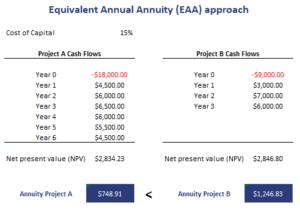# Equivalent Annual Annuity approach

The Equivalent Annual Annuity (EAA) approach or equivalent annual cash flow approach is a capital budgeting approach. It is used to compare mutually exclusive projects with unequal lives. This method is an alternative to the Least Common Multiple of Lives approach. The advantage of the Equivalent Annual Annuity approach however, is that it is simpler than the least common multiple of lives method.

On this page, we discuss the steps involved when applying the EAA approach. We also apply the method using a numerical example. An equivalent annual cost Excel template is available for download at the bottom of the page.

## Equivalent Annual Annuity Steps

There is no single equivalent annual cost formula. Rather, applying the equivalent annual annuity method requires that we follow three steps

1. First, we calculate each projects net present value (NPV)
2. Next, we find the annuity with the present value equal to the project’s NPV over its life at the weighted average cost of capital (WACC)
3. We select the project with the highest EAA.

## Equivalent annual annuity calculator

Let’s apply the methodology using a numerical example. This will immediately make clear how one can easily apply the methodology using spreadsheet software. The following table reports data on two potential projects and applies the EAA approach. The equivalent annual annuity Excel template calculator is available for download below.Thus, all we need to do is to calculate the net present values of both projects and then calculate the associated annuity. The project with the highest annuity value is the project we should choose.

## Summary

We discussed how to calculate equivalent annual annuity. The equivalent uniform annual cost method is three step process that allows managers to compare mutually exclusive project with unequal lives. The approach is easier to apply than the least common multiples of lives method.